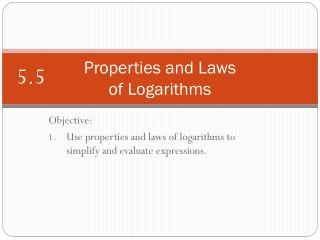DownloadDownload PresentationProperties and Laws of Logarithms

# Properties and Laws of Logarithms

Download Presentation## Properties and Laws of Logarithms

- - - - - - - - - - - - - - - - - - - - - - - - - - - E N D - - - - - - - - - - - - - - - - - - - - - - - - - - -
##### Presentation Transcript

1. Properties and Lawsof Logarithms 5.5 Objective: Use properties and laws of logarithms to simplify and evaluate expressions.

2. Basic Properties of Logarithms

3. Example #1Solving with the Basic Properties • Use the basic properties of logarithms to solve the equations below. A power with base 10 is the inverse of the common log. Since they are inverses they “undo” each other and we are left with the resulting expression. Don’t forget when something is done to one side of an equation, it must also be done to the other.

4. Example #1Solving with the Basic Properties • Use the basic properties of logarithms to solve the equations below. Just like common logs, natural logs have an inverse as well, but with powers of base e.

5. Product Law of Logarithms

6. Example #2 • Use the Product Law of Logarithms to evaluate each logarithm.

7. Example #2 • Use the Product Law of Logarithms to evaluate each logarithm.

8. Example #2 • Use the Product Law of Logarithms to evaluate each logarithm.

9. Quotient Law of Logarithms

10. Example #3 • Use the Quotient Law of Logarithms to evaluate each logarithm.

11. Example #3 • Use the Quotient Law of Logarithms to evaluate each logarithm.

12. Power Law of Logarithms

13. Example #4 • Use the Power Law of Logarithms to evaluate each logarithm.

14. Example #4 • Use the Power Law of Logarithms to evaluate each logarithm.

15. Example #5Simplifying Expressions • Write each expression as a single logarithm. When condensing logarithms into a single logarithm, you must use the properties of logarithms backwards. Always bring coefficients up as exponents first, then combine addition and subtraction by multiplication and division.

16. Example #5Simplifying Expressions • Write each expression as a single logarithm. You cannot change the subtraction into division because they both must be logarithms.

17. Example #5Simplifying Expressions • Write each expression as a single logarithm. Alternatively, this problem can also be solve by expanding the expression first before condensing.

18. Example #5Simplifying Expressions • Write each expression as a single logarithm. Like the previous problem, this one can also be solved by introducing a logarithm first, but this time use the fact that:

19. Example #5Simplifying Expressions • Write each expression as a single logarithm. This problem could have been solved by expanding first as well:

20. Example #6Simplifying by Substitution • Write the expression in terms of u and v given Expand the expression first, the substitute.

21. Example #6Simplifying by Substitution • Write the expression in terms of u and v given